GRE Subject Test: Math : Evaluating Logarithms

Example Questions

Example Question #1 : Logarithms

Solve: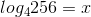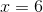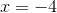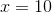Explanation:

Step 1: Re-write the log equation as an exponential equation. To do this, take the base of the log function and raise it to the number on the right side of the equal sign. This new exponent is equal to the number to the right of the log base.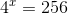Step 2: Re-write the right hand side as a power of 4..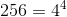Step 3: Re-write the equation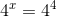Step 4: We have the same base, so we can equal the exponents..Example Question #2 : Logarithms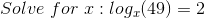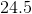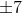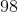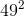Explanation:

In order to solve for x, we must first rewrite the log in exponential form.

Every log is written in the below general form: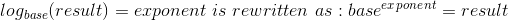In this case we have: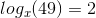This becomes: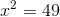We can solve this by taking the square root of both sides: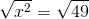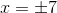Example Question #3 : Logarithms

Solve for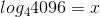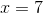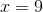Explanation:

Use rules of logarithms...

Take the base of the log and raise it to the number on the right side of the equal sign (which becomes the exponent):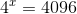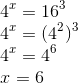Example Question #4 : Logarithms

Evaluate: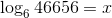Explanation:

Step 1: Write the expression in exponential form...

Given: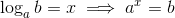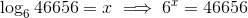Step 2: Convert the right hand side into a power of 6..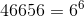Step 3: Re-write the equations...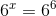Since the bases are equal, taking log of both sides will cancel them.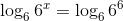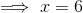Example Question #5 : Logarithms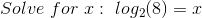Explanation: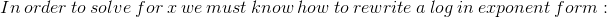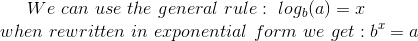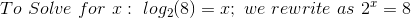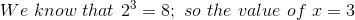All GRE Subject Test: Math Resources